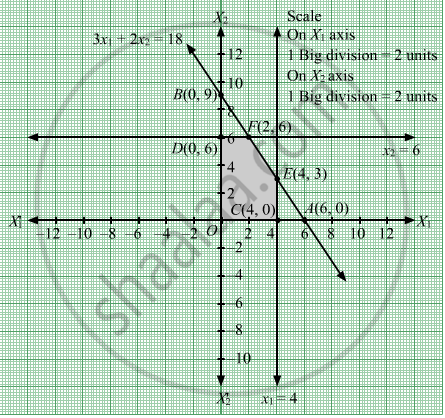# By Graphical Method, the Solution of Linear Programming Problem Maximize Z = 3 X 1 + 5 X 2 Subject T O 3 X 1 + 2 X 2 ≤ 18 X 1 ≤ 4 X 2 ≤ 6 X 1 ≥ 0 , X 2 ≥ 0 , I S - Mathematics

MCQ
Sum

By graphical method, the solution of linear programming problem

$\text{Maximize}\text{ Z }= 3 x_1 + 5 x_2$
$\text{ Subject } to \text{ 3 } x_1 + 2 x_2 \leq 18$
$x_1 \leq 4$
$x_2 \leq 6$
$x_1 \geq 0, x_2 \geq 0, \text{ is }$

#### Options

• x1 = 2, x2 = 0, Z = 6

• x1 = 2, x2 = 6, Z = 36

• x1 = 4, x2 = 3, Z = 27

• x1 = 4, x2 = 6, Z = 42

#### Solution

x1 = 2, x2 = 6, Z = 36

We need to maximize the function Z = 3x1 + 5x2
First, we will convert the given inequations into equations, we obtain the following equations:  3x1 + 2x2 = 18, x1 = 4, x2 = 6, x1 = 0 and x2 = 0

Region represented by 3x1 + 2x2 ≤ 18:
The line 3x1 + 2x2 = 18 meets the coordinate axes at A(6, 0) and B(0, 9) respectively. By joining these points we obtain the line 3x1 + 2x2 = 18.
Clearly (0,0)  satisfies the inequation 3x1 + 2x2 = 18 .So,the region in the plane which contain the origin represents the solution set of the inequation  3x1 + 2x2 = 18.

Region represented by x1 ≤ 4:
The line x1 = 4 is the line that passes through C(4, 0) and is parallel to the Y axis. The region to the left of the line x1 = 4 will satisfy the inequation x1 ≤ 4.
Region represented by x2 ≤ 6:
The line x2 = 6 is the line that passes through D(0, 6) and is parallel to the X axis. The region below the line x2 = 6 will satisfy the inequation x2 ≤ 6.

Region represented by x1 ≥ 0 and x2 ≥ 0:
Since, every point in the first quadrant satisfies these inequations. So, the first quadrant is the region represented by the inequations x1 ≥ 0 and x2 ≥ 0.

The feasible region determined by the system of constraints, 3x1 + 2x2 ≤ 18, x1 ≤ 4x2 ≤ 6, x1 ≥ 0, and x2 ≥ 0, are as followsCorner points are O(0, 0), D(0, 6), F(2, 6), E(4, 3) and C(4, 0).
The values of the objective function at these points are given in the following table

 Points Value of Z O(0, 0) 3(0)+5(0) = 0 D(0, 6) 3(0)+5(6) = 30 F(2, 6) 3(2)+5(6) = 36 E(4, 3) 3(4)+5(3) = 27 C(4, 0) 3(4)+5(0) = 12

We see that the maximum value of the objective function Z is 36 which is at F(2, 6).

Concept: Graphical Method of Solving Linear Programming Problems
Is there an error in this question or solution?

#### APPEARS IN

RD Sharma Class 12 Maths
Chapter 30 Linear programming
MCQ | Q 13 | Page 68